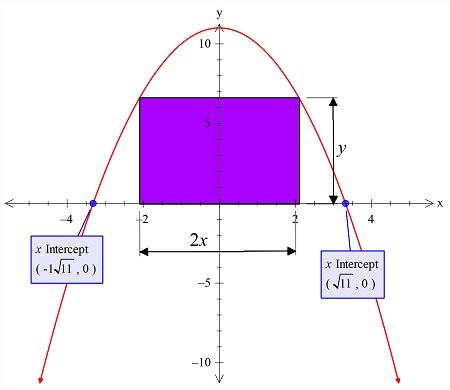# A rectangle is inscribed with its base on the x-axis and its upper corners on the parabola y = 11...

## Question:

A rectangle is inscribed with its base on the {eq}x {/eq}-axis and its upper corners on the parabola {eq}y = 11 - x^{2} {/eq}.

What are the dimensions of such a rectangle with the greatest possible area?

## Finding Minima & Maxima:

In the curve that the bounded with the coordinate axes can have the geometrical shape inscribed in it and that geometrical shape can have the maximum or the minimum area, that is found using the differentiation method.

We have to find the curve with the given equation and then we will inscribe the rectangle inside it. Now the rectangle area is given in the color shown in the figure below:Here the rectangle is having the length 2x and the width of y.

Now the area of this rectangle is

{eq}A=2xy \\ =2x (11 - x^{2})\\ {/eq}

Now to maximize this area the first derivative is put zero and the critical point is found next, as follows:

{eq}A'=0\\ \Rightarrow \frac{d}{dx}\left(2x\:\left(11\:-\:x^2\right)\right)=0\\ \Rightarrow 2\left(\frac{d}{dx}\left(x\right)\left(11-x^2\right)+\frac{d}{dx}\left(11-x^2\right)x\right)=0\\ \Rightarrow 2\left(11-3x^2\right)=0\\ \Rightarrow x=\sqrt{\frac{11}{3}}\\ {/eq}

So at this x value, the rectangle will have the maximum area.

And hence the dimension of the rectangle is:

{eq}Length=2x= 2\sqrt{\frac{11}{3}}\\ Width=y= (11 - (\sqrt{\frac{11}{3}})^{2}) {/eq}